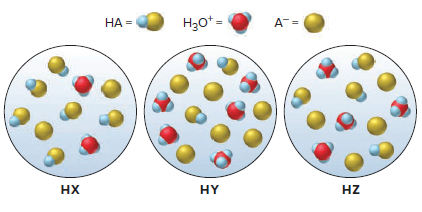⚠️Our tutors found the solution shown to be helpful for the problem you're searching for. We don't have the exact solution yet.

# Solution: The following scene represent three weak acid HA (where A = X, Y, or Z) dissolved in water (H2O is not shown):(e) If equimolar amounts of the sodium salts of the acids (NaX, NaY, and NaZ) were dissolved in water, which solution would have the highest pOH? The lowest pH?

###### Problem

The following scene represent three weak acid HA (where A = X, Y, or Z) dissolved in water (H2O is not shown):

(e) If equimolar amounts of the sodium salts of the acids (NaX, NaY, and NaZ) were dissolved in water, which solution would have the highest pOH? The lowest pH?